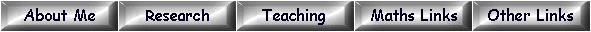Elements of order 5 in the Tate-Shafarevich group

We list elements of order 5 in the Tate-Shafarevich group for elliptic curves E over Q. To compile this data we started from Cremona's list of elliptic curves over Q with "analytic order of Sha" greater than 1. The data is available at

http://www.warwick.ac.uk/~masgaj/ftp/data/

For each elliptic curve over Q with analytic order of Sha divisible by 5 we expect to find a subgroup of Sha isomorphic to Z/5Z x Z/5Z. We have found such a subgroup in all cases up to conductor 350000 (the current limit of Cremona's tables).

The first line of each entry specifies an elliptic curve E over Q. This data is taken directly from Cremona's tables. The quantities listed are the conductor, the isogeny class, the number in the isogeny class, the coefficients [a1,a2, a3,a4,a6] of a minimal Weierstrass equation, the Mordell-Weil rank, the order of the torsion subgroup, and finally the analytic order of Sha. The next line gives some data relating to how the example was computed. We then list (5k - 1)/2 genus one models of degree 5, representing a subgroup of the 5-Selmer group of E isomorphic to (Z/5Z)k where k = 2 or 4 (the latter so far only in the case 165066d3). Each genus one model corresponds to a non-zero element of the subgroup and its inverse.

We recall that a genus one model of degree 5 is a skew-symmetric matrix ϕ of linear forms in 5 variables. The corresponding curve is defined by the 4 × 4 Pfaffians of ϕ. We convert ϕ to a sequence of 50 coefficients by taking the linear forms in the order

ϕ12, ϕ13, ϕ14, ϕ15, ϕ23, ϕ24, ϕ25, ϕ34, ϕ35, ϕ45.

We have checked that each subgroup of the Selmer group listed has trivial intersection with the image of the Mordell-Weil group. (In all examples so far the Mordell-Weil rank is 0 or 1.) Therefore each curve listed is a counterexample to the Hasse Principle.

The genus one models listed have been minimised and reduced as described in . We have also arranged that the reduction mod p at each prime p is geometrically (i.e. over the algebraic closure of Fp) either an irreducible curve of degree 5, or (in some cases where the Tamagawa number of E at p is divisible by 5) the union of a rational normal curve of degree 4 and a line. In particular there are always smooth Fp-points on the reduction mod p, and this guarantees local solubility.

These tables were constructed using the following methods :-

• In most examples where E admits a rational 5-isogeny, the required elements of Sha are in the kernel of the map induced by the 5-isogeny. Explicit equations for each element of Sha are then found by descent by 5-isogeny, as described in  and . In cases where E is a quadratic twist by d of one of the curves Cλ defined in , we record the values of λ and d.
• In most examples where E does not admit a rational 5-isogeny, we find that the 5-torsion of Sha is visible (in the sense of Mazur ) in an abelian surface isogenous to E × F, where F is an elliptic curve over Q with Mordell-Weil rank 2 or 3. We specify F in the second row of each entry, giving the data in the same format as for E. In some cases F is beyond the range of Cremona's tables. Explicit equations for each element of Sha are then computed using the Hessian , and related covariants , as contributed to MAGMA  by the author. The same formulae allows us to compute elements of Sha for E from those for E' where E and E' are related by an isogeny of degree coprime to 5.
• In the remaining examples we used full 5-descent, as described in  and , and contributed to MAGMA  by the author. If possible we first used visibility (usually with F a curve of rank 1) to find some elements of Sha, and then used 5-descent to "add" these examples. This left a comparatively small number of examples where we needed to compute the S-units in a degree 24 number field. The latter were computed by Steve Donnelly in September 2014.

A similar study of elements of Sha of order 3 is here.

References

Last updated 16th February 2015